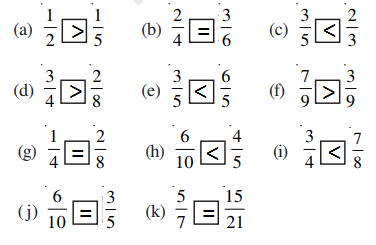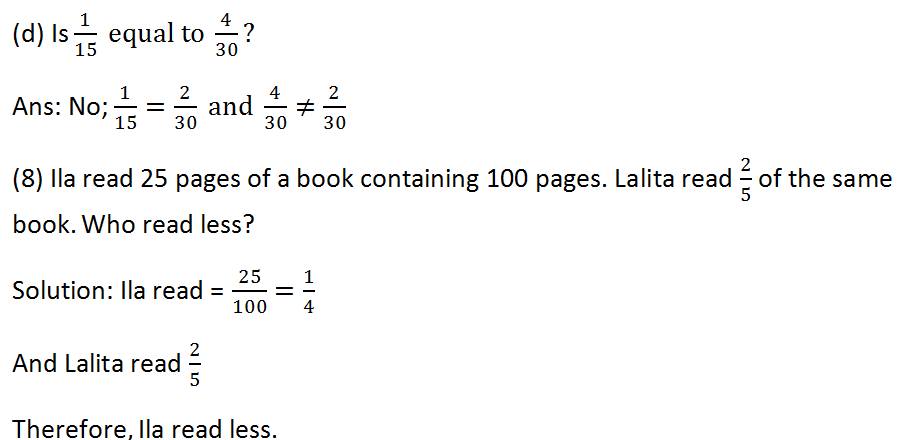# RS Aggarwal Vikash Aggarwal Class 2 Activity Book Composite Mathematics Activity Sheet 71 To Sheet 75 Solution

## Activity Sheet – 71

#### Count the number of horizontal lines, vertical lines and slanting lines in each of the following figures:## Activity Sheet – 72

#### Look at the numbers in different shapes given above and answer the following questions:(1) The smallest number inside a circle is 123.

(2) The greatest number inside a rectangle is 875.

(3) The smallest number inside a triangle is 469.

(4) The smallest number inside a square is 234.

(5) The greatest number inside a circle is 321.

(6) The smallest number inside a rectangle is 578.

(7) The greatest number inside a triangle is 964.

(8) The greatest numbers inside a square is 432.

(9) Arrange the numbers in the circles in an ascending order:

Ans: 123, 132, 213, 231, 312, 314.

(10) Arrange the numbers in the triangles in a descending order:

Ans: 964, 946, 694, 649, 496, 469.

## Activity Sheet – 73

(1) How many faces does each of the following shapes have?

• Sphere – 0
• Cone – 1
• Cylinder – 2
• Cube – 6

(2) Tick the shapes having only one curved face.

• Sphere
• Cone
• Cylinder

(3) Tick the shapes which can roll on an inclined plane.

• Sphere
• Cylinder

(4) Tick the shapes which have at least one circular edge.

• Cone

(5) Tick the shapes with no vertex.

• Sphere
• Cylinder

## Activity Sheet – 74

#### State whether the given statement is true or false:

(1) A triangle has four vertices. – False

(2) A cone has one edge. – False

(3) Each face of a cube is square – True

(4) We use a ruler to draw a straight line. – True

(5) All the sides of a rectangle are equal. – False

(6) A plane paper represents a cuboid. – True

(7) A cuboid has 6 vertices and 12 edges. – False

(8) A cylinder has two vertices. – False

(9) A cone has two faces-one plane and one curved. – False

(10) A sphere has no vertex. – True

(11) All the edges of cuboid are equal. – False

## Activity Sheet – 75

#### Write the correct time shown by each clock in two different ways:####Updated: September 4, 2018 — 11:46 am